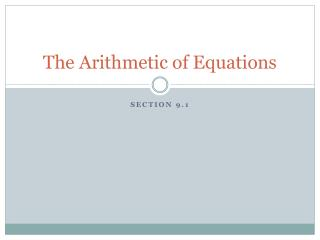Download PresentationThe Arithmetic of Equations

# The Arithmetic of Equations

Download Presentation## The Arithmetic of Equations

- - - - - - - - - - - - - - - - - - - - - - - - - - - E N D - - - - - - - - - - - - - - - - - - - - - - - - - - -
##### Presentation Transcript

1. The Arithmetic of Equations Section 9.1

2. Interpreting Chemical Equations In terms of moles: Example Equation: 2H2 + O2 2H2O 2 mol H2 + 1 mol O2 = 2 mol H2O

3. Interpreting Chemical Equations In terms of particles: • You know that Avogradro’s Number relates particles and moles (6.02 x 1023 particles = 1 mole) • Example Equation: 2H2 + O2 2H2O 2 mol H2 x 6.02 x 1023 particles + 1 mol 1 mol O2 x 6.02 x 1023 particles = 1 mol 2 mol H2 x 6.02 x 1023 particles 1 mol 1.204 x 1024 particles of H2 + 6.02 x 1023 particles of O2 = 1.204 x 1024 particles of H2O

4. Interpreting Chemical Equations In terms of mass: • 1 mole = molar mass of element or compound • Example Equation: 2H2 + O2 2H2O 2 mol H2 x 2.0158 grams + 1 mol 1 mol O2 x 31.998 grams = 1 mol 2 mol H2 x 18.0148 grams 1 mol 4.0316 grams of H2 + 31.998 grams of O2 = 36.296 grams of H2O

5. Interpreting Chemical Equations In terms of volume: • 1 mole = 22.4 Liters • Example Equation: 2H2 + O2 2H2O 2 mol H2 x 22.4 L + 1 mol 1 mol O2 x 22.4 L = 1 mol 2 mol H2 x 22.4 L 1 mol 44.8 L of H2 + 22.4 L of O2 = 44.8 L of H2O

6. After reading Section 9.1, you should know: How to balance a chemical equation How to calculate particles, moles, volume and mass from a balanced equation